Domain adaption 是目前在 machine learning 比較新的問題，是希望可以讓 network 學到比較跨領域的 features ，進而使訓練出來的模型可以應用在不同 domain 的資料上。

Introduction

• 作者引入了 CORAL 這一方法，並且將其擴展成一 differentiable loss function。
• 作者藉由 CORAL loss 來做 unsupervised learning，並達到了 state-of-the-art 的 performance。
• CORAL loss 十分簡單並且可以輕易地整合至一般的神經網路中。

Architecture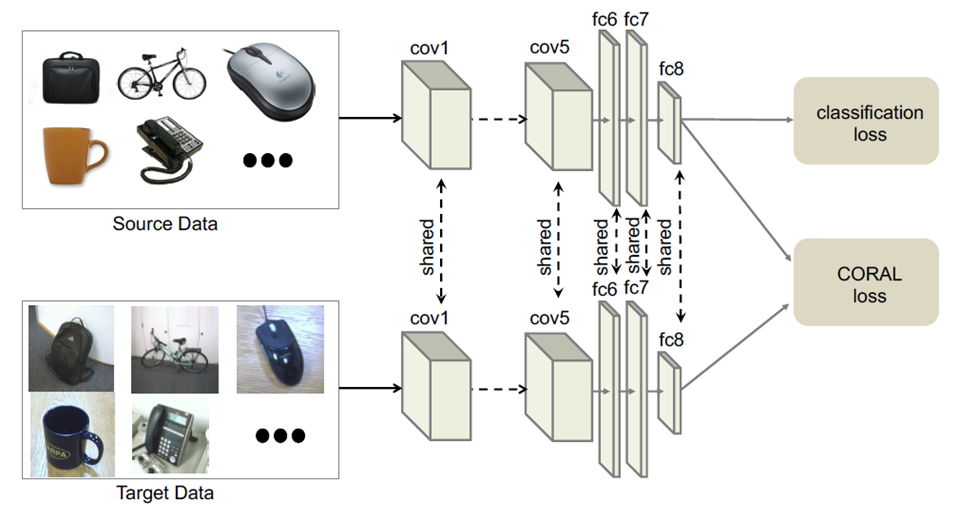作者將 CORAL loss 加進一分類問題的網路架構中。 

source data & target data 各自經過一 shared weight 的 networks 之後會有兩個 output，其中:

• source task 會算一個 classification loss
• source 的 fc8 及 target 的 fc8 會再拿來算 CORAL loss

$$loss = l_{CLASS} + \lambda l_{CORAL}$$

CORAL Loss

We define the CORAL loss as the distance between the second-order statistics
(covariances) of the source and target features.

Forward

$$l_{CORAL} = \frac {1} {4d^{2}} \lVert C_S - C_T \rVert ^{2}_F$$

$$C_S$$, $$C_T$$ 為 source, target 的 covariance matrix，其定義如下:

$$\ C_S = \frac{1}{n_S - 1}(D^{\intercal}_S D_S-\frac {1} {n_S} (\textbf{l}^{\intercal}D_S )^\intercal(\textbf{l}^\intercal D_S))$$

$$\ C_T = \frac{1}{n_T - 1}(D^{\intercal}_T D_T-\frac {1} {n_T} (\textbf{l}^{\intercal}D_T )^\intercal(\textbf{l}^\intercal D_T))$$

$$\ \frac{\partial l_{CORAL}}{\partial D^{ij}_S}=\frac {1}{d^{2}(n_S-1)} (D^{\intercal}_S-\frac{1}{n_S}((\textbf{l}^{\intercal}D_S )^\intercal\textbf{l}^{\intercal})^{\intercal}(C_S - C_T))^{ij}$$

$$\ \frac{\partial l_{CORAL}}{\partial D^{ij}_T}=-\frac {1}{d^{2}(n_T-1)} (D^{\intercal}_T-\frac{1}{n_T}((\textbf{l}^{\intercal}D_T )^\intercal\textbf{l}^{\intercal})^{\intercal}(C_S - C_T))^{ij}$$

Experiment

• Amazon 就是去背的圖片(背景都是白色的)
• DSLR 就是用單眼拍的圖片(背景就是真實場景的背景)
• Webcam 跟 DSLR 很相近，差別比較大的部分是 webcam 的畫質比較差，有的還有色偏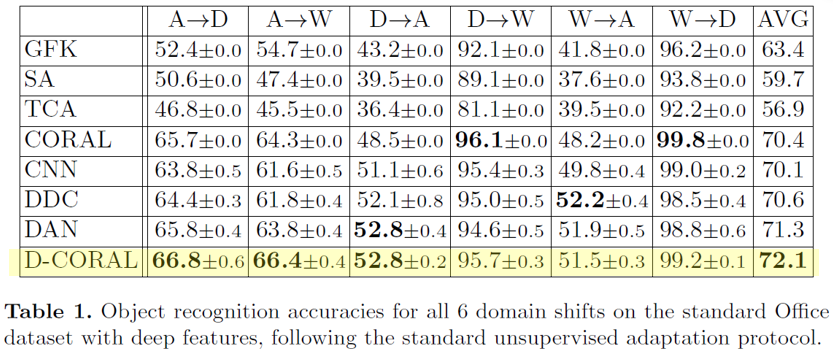各種方法比較圖。螢光的是作者的方法。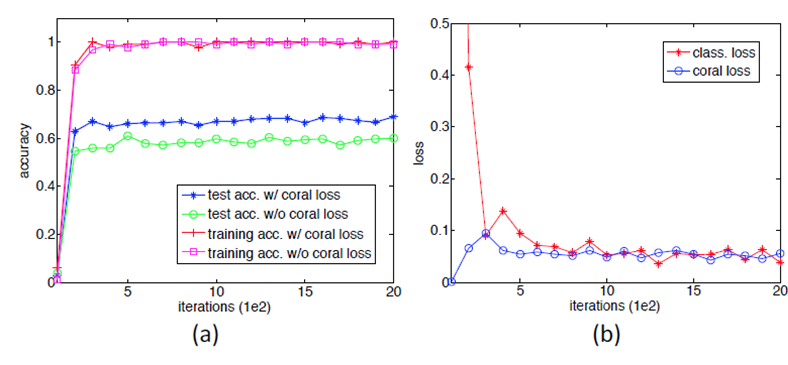Amazon → Webcam 的詳細結果圖。

Implementation

Forward 的部分大概如下:

Backward 則如下: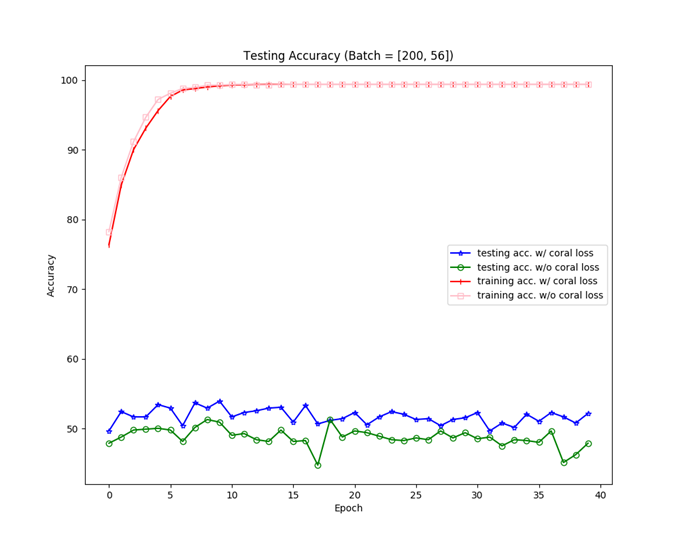我做出來的 Amazon → Webcam 的詳細結果圖。

Update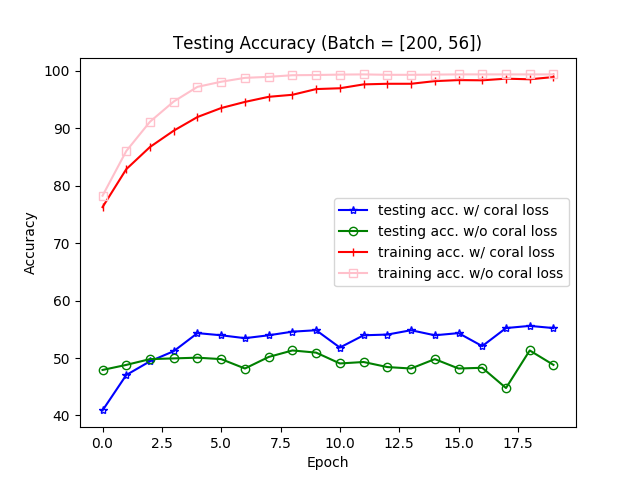修正過後的結果。

 Sun, B., Saenko, K.: Deep CORAL: Correlation Alignment for Deep Domain Adaptation. In: ECCV (2016)
 Sun, B., Feng, J., Saenko, K.: Return of frustratingly easy domain adaptation. In: AAAI (2016)